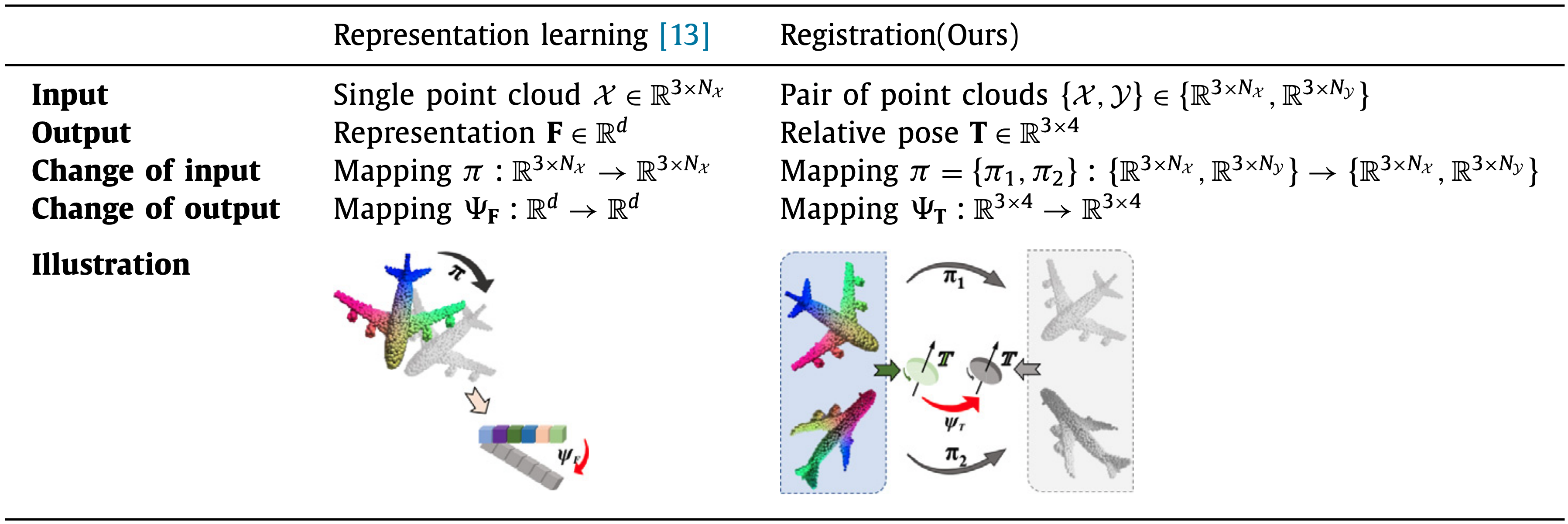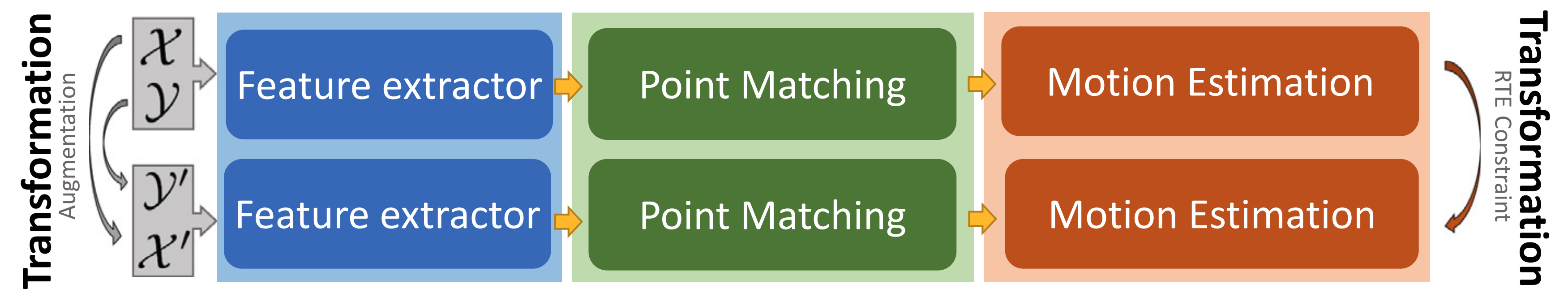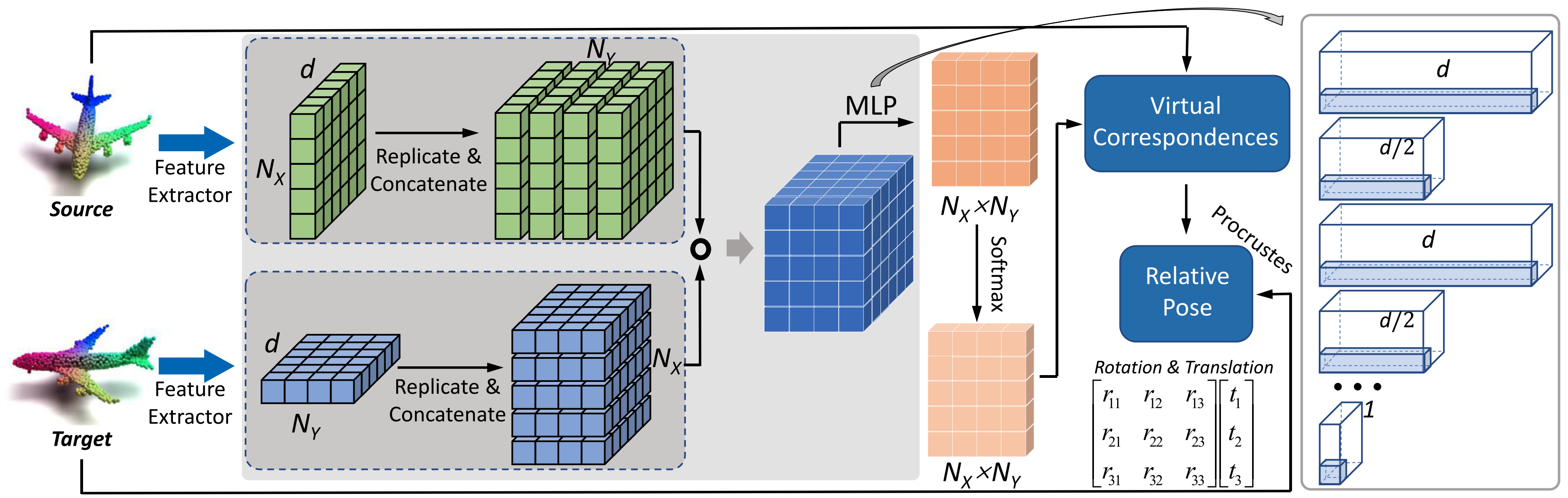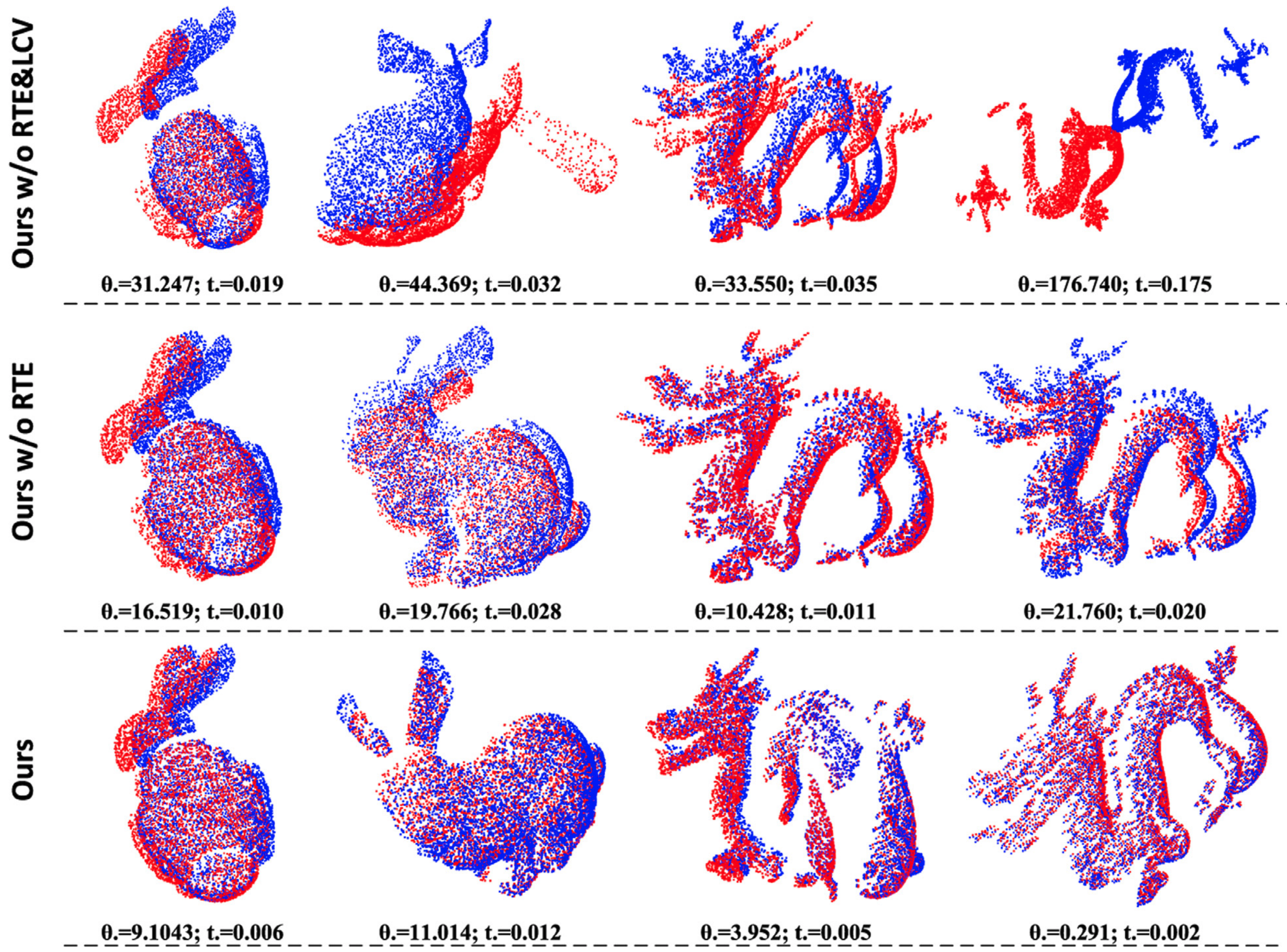## Self-supervised rigid transformation equivariance for accurate 3D point cloud registration

#### Pattern Recognition

###### Zhiyuan Zhang1, Jiadai Sun1, Yuchao Dai1, Dingfu Zhou2, Xibin Song2, Mingyi He1

1Northwestern Polytechnical University    2Baidu Inc

### Abstract

Transformation equivariance has been widely investigated in 3D point cloud representation learning for more informative descriptors, which formulates the change of the representation with respect to the transformation of the input point clouds explicitly. In this paper, we extend this property to the task of 3D point cloud registration and propose a rigid transformation equivariance (RTE) for accurate 3D point cloud registration. Specifically, RTE formulates the change of the relative pose explicitly with respect to the rigid transformation of the input point clouds. To exploit RTE, we adopt a Siamese structure network with two shared registration branches. One focuses on the input pair of point clouds, and the other one focuses on the new pair achieved by applying two random rigid transformations to the input point clouds respectively. Since the change of the two output relative poses has been predicted according to RTE, a new additional self-supervised loss is obtained to supervise the training. This general network structure can be integrated with most learning-based point cloud registration frameworks easily to improve the performance. Our method adopts the state-of-the-art virtual point-based pipelines as our shared branches, in which we propose a data-driven matching based on learned cost volume (LCV) rather than traditional hand-crafted matching strategies. Experimental evaluations on both synthetic datasets and real datasets validate the effectiveness of our proposed framework.

### Contribution

• We construct a dedicated RTE in 3D point cloud registration and design a Siamese network structure instead of the traditional “single branch” network. Our RTE structure can be integrated with the learning-based frameworks easily to improve the registration performance.
• We propose to learn the matching matrix from the LCV instead of the hand-crafted matching strategy, which is more effective and efficient.
• Remarkable performance on several datasets topping the stateof-the-art methods proves the effectiveness of our proposed method.

### MotivationComparison of the equivariance property in 3D point cloud representation learning task and registration task.

### Network ArchitectureIllustration of our proposed 3D point cloud registration framework (RTE-structure). First, we augment the input point clouds $\mathcal{X}$ and $\mathcal{Y}$ with random rigid transformations to $\mathcal{X}^\prime$ and $\mathcal{Y}^\prime$. Then, two shared branches are used to estimate the relative poses $\mathbf{T}$ and $\mathbf{T}^\prime$ between $\{\mathcal{X},\mathcal{Y}\}$ and $\{\mathcal{X}^\prime,\mathcal{Y}^\prime\}$ respectively. Each branch network consists of feature extractor, point matching, and motion estimation. The augmentation operation and the inherent RTE constraint, connect the input and output of the two branches respectively, where a closed-loop is constructed. This explicit correlation provides a self-supervised loss function without any extra ground truth information. This RTEstructure can be integrated into other learning-based point cloud registration easily.Illustration of the branch registration network architecture. First, we extract the point features for the source and target point clouds $\mathcal{X}$ and $\mathcal{Y}$. Then, in the matching stage, we construct the LCV by replicating and concatenating the features. And the subsequent MLP and row-wise softmax are applied to regress the LCV to the matching matrix. The virtual corresponding points to $\mathcal{X}$ are obtained by using matching matrix to perform the weighted average on $\mathcal{Y}$. Finally, the Procrustes algorithm is used to estimate the rotation matrix $\mathbf{R}$ and translation vector $\mathbf{t}$. Right part shows the detailed matching matrix learning process.

### Ablation AnalyzeVisualization of Stanford scan data. We provide registration results (i.e. corresponding angular error θ. and translation vector error t.) of Bunny and Dragon. And the complete method is much better than ours w/o RTE and ours w/o RTE&LCV considerably.

### Citation

@ARTICLE{zhang_rte_pr_2022,
title={Self-supervised rigid transformation equivariance for accurate 3D point cloud registration},
author={Zhiyuan Zhang and Jiadai Sun and Yuchao Dai and Dingfu Zhou and Xibin Song and Mingyi He},
journal={Pattern Recognition},
volume    = {130},
pages     = {108784},
year      = {2022}}